# BAYESIAN NETWORKS Bayesian Network Motivation We want a

• Slides: 31BAYESIAN NETWORKS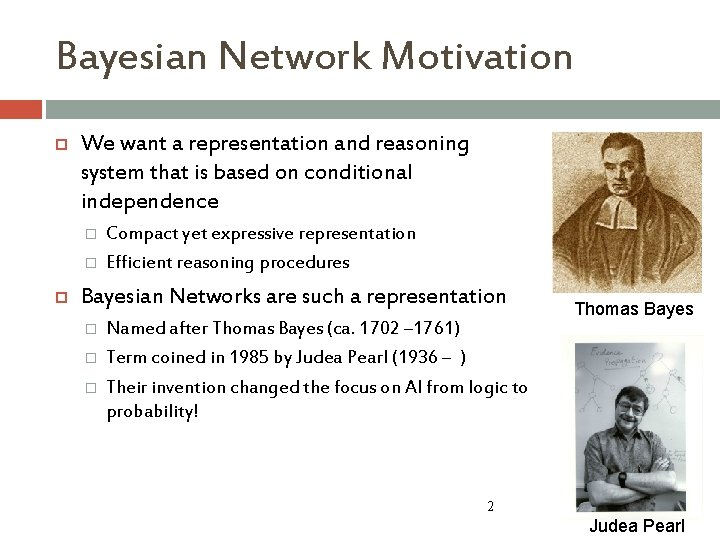Bayesian Network Motivation We want a representation and reasoning system that is based on conditional independence � � Compact yet expressive representation Efficient reasoning procedures Bayesian Networks are such a representation � � � Named after Thomas Bayes (ca. 1702 – 1761) Term coined in 1985 by Judea Pearl (1936 – ) Their invention changed the focus on AI from logic to probability! Thomas Bayes 2 Judea Pearl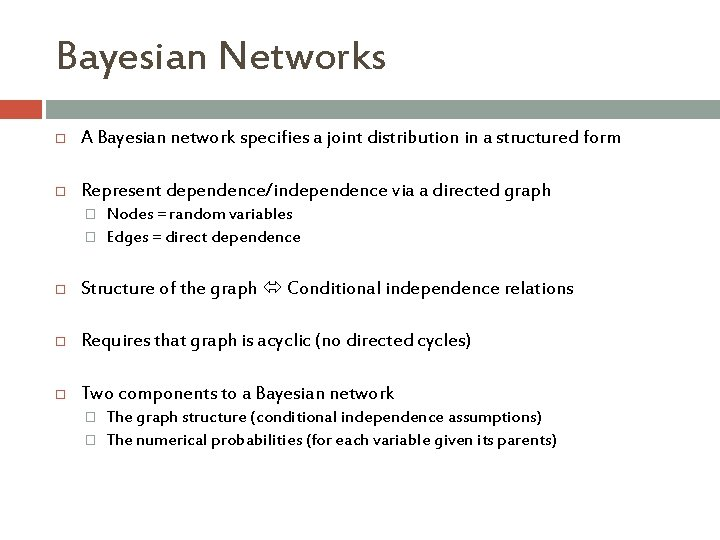Bayesian Networks A Bayesian network specifies a joint distribution in a structured form Represent dependence/independence via a directed graph � � Nodes = random variables Edges = direct dependence Structure of the graph Conditional independence relations Requires that graph is acyclic (no directed cycles) Two components to a Bayesian network � � The graph structure (conditional independence assumptions) The numerical probabilities (for each variable given its parents)Bayesian Networks General form: The full joint distribution The graph-structured approximation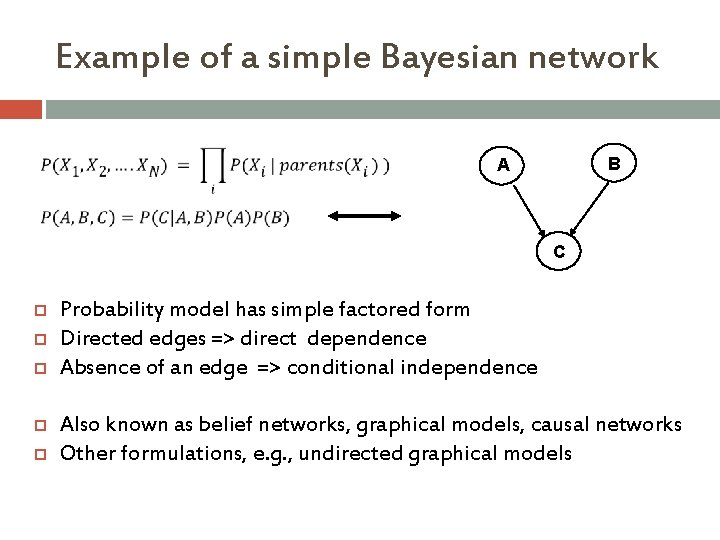Example of a simple Bayesian network B A C Probability model has simple factored form Directed edges => direct dependence Absence of an edge => conditional independence Also known as belief networks, graphical models, causal networks Other formulations, e. g. , undirected graphical models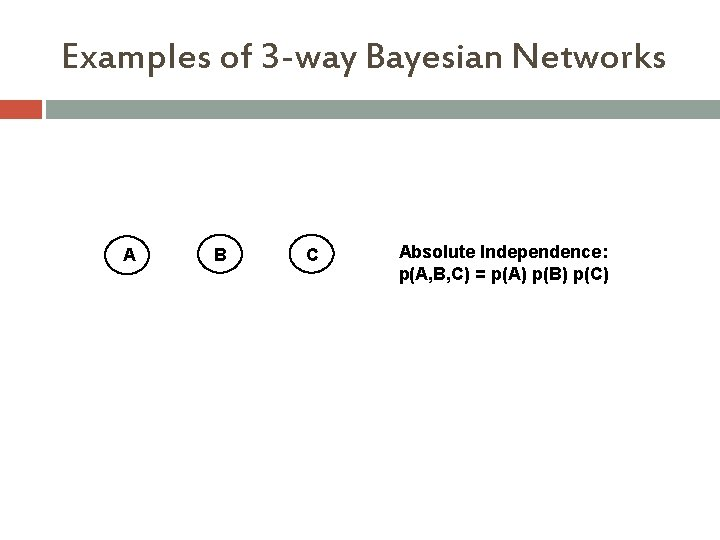Examples of 3 -way Bayesian Networks A B C Absolute Independence: p(A, B, C) = p(A) p(B) p(C)Examples of 3 -way Bayesian Networks A B CExamples of 3 -way Bayesian Networks A B C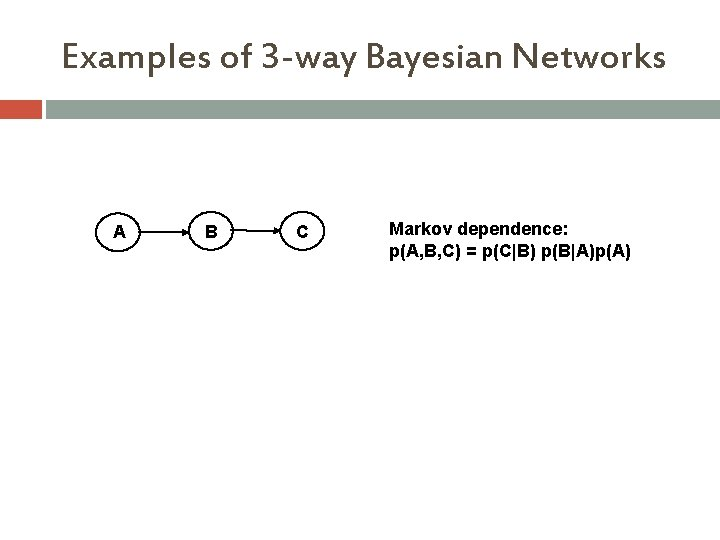Examples of 3 -way Bayesian Networks A B C Markov dependence: p(A, B, C) = p(C|B) p(B|A)p(A)The Alarm Example You have a new burglar alarm installed It is reliable about detecting burglary, but responds to minor earthquakes Two neighbors (John, Mary) promise to call you at work when they hear the alarm � John always calls when hears alarm, but confuses alarm with phone ringing (and calls then also) � Mary likes loud music and sometimes misses alarm! Given evidence about who has and hasn’t called, estimate the probability of a burglary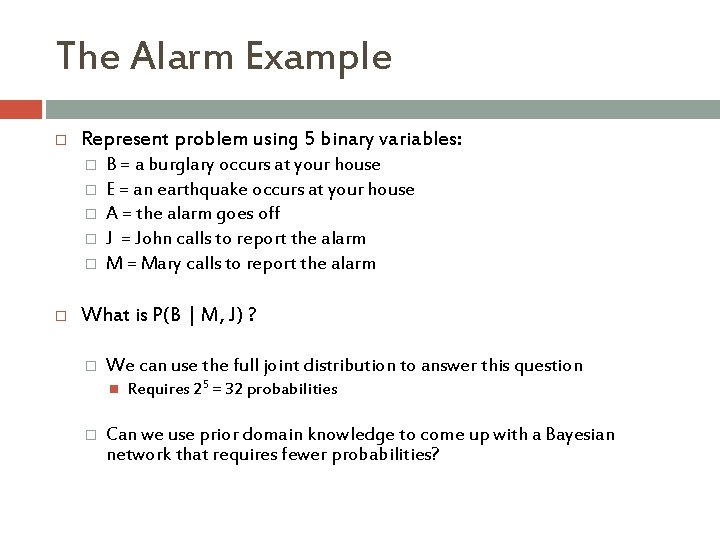The Alarm Example Represent problem using 5 binary variables: � � � B = a burglary occurs at your house E = an earthquake occurs at your house A = the alarm goes off J = John calls to report the alarm M = Mary calls to report the alarm What is P(B | M, J) ? � We can use the full joint distribution to answer this question � Requires 25 = 32 probabilities Can we use prior domain knowledge to come up with a Bayesian network that requires fewer probabilities?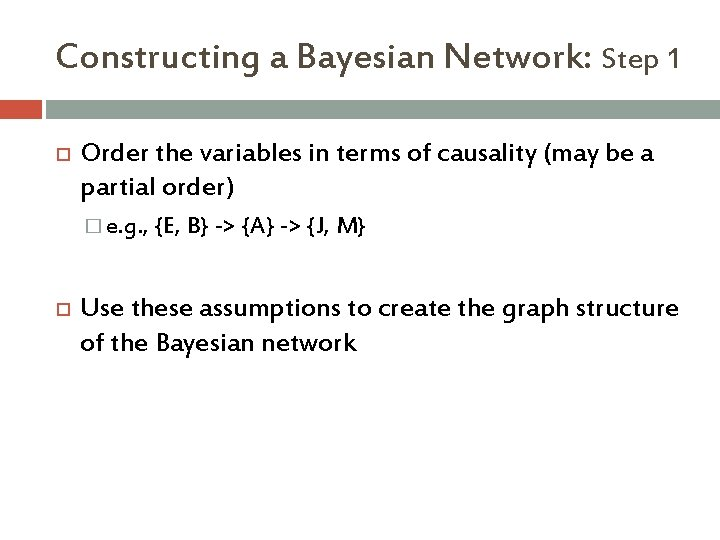Constructing a Bayesian Network: Step 1 Order the variables in terms of causality (may be a partial order) � e. g. , {E, B} -> {A} -> {J, M} Use these assumptions to create the graph structure of the Bayesian network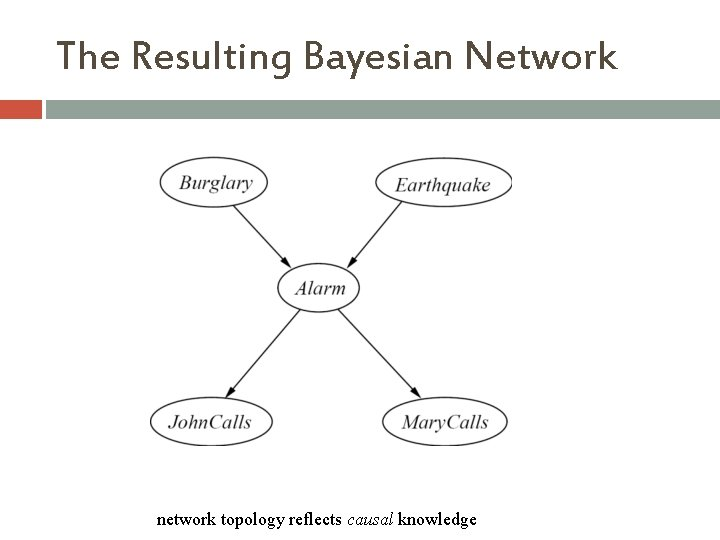The Resulting Bayesian Network network topology reflects causal knowledge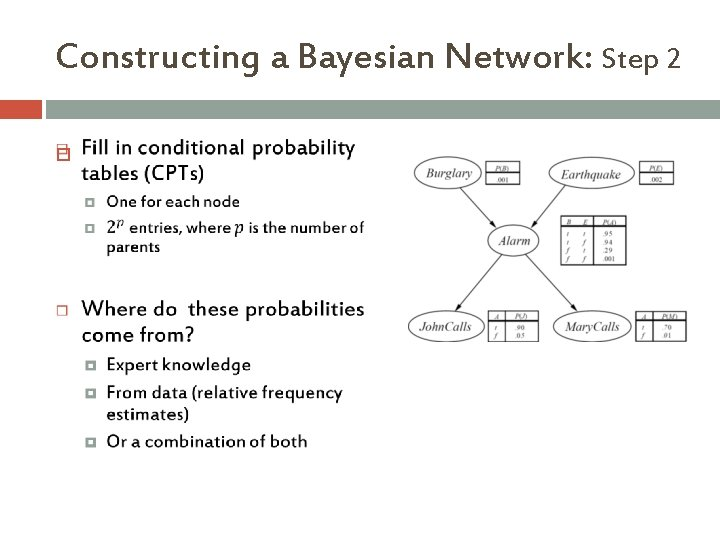Constructing a Bayesian Network: Step 2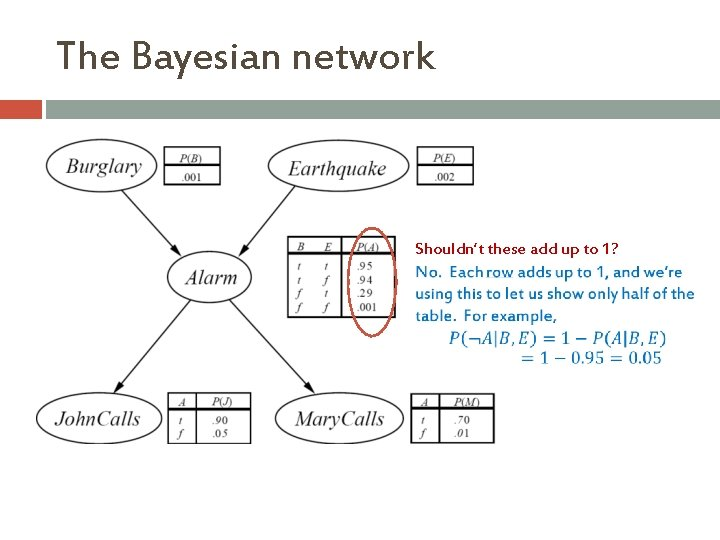The Bayesian network Shouldn’t these add up to 1?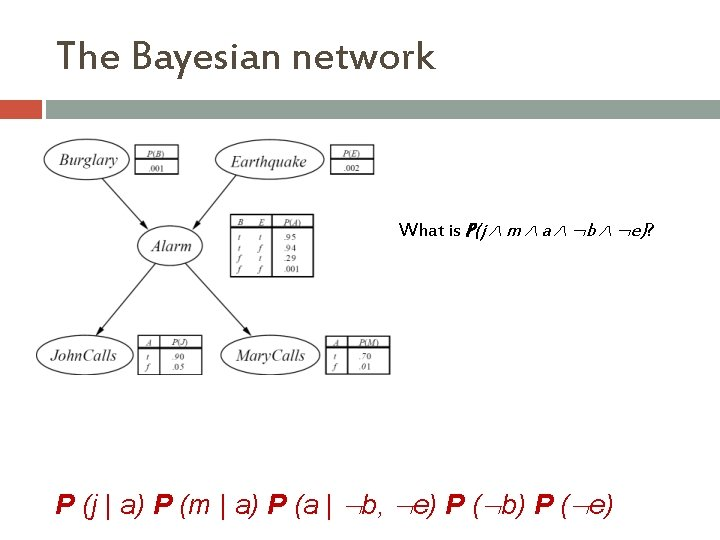The Bayesian network What is P(j m a b e)? P (j | a) P (m | a) P (a | b, e) P ( b) P ( e)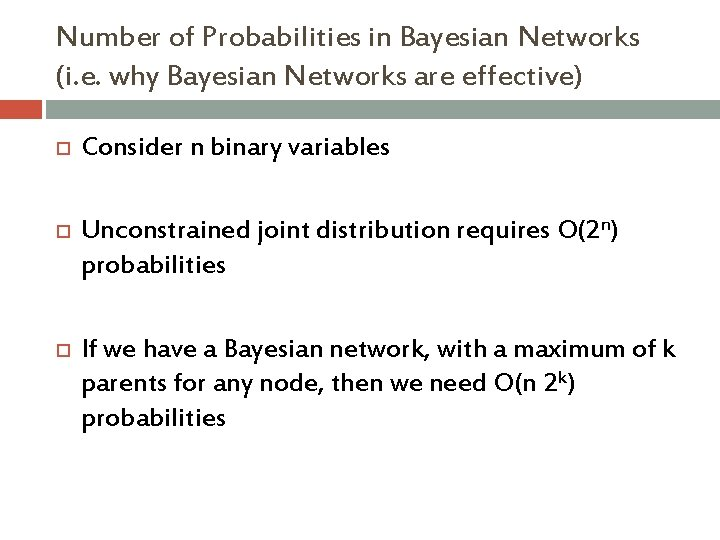Number of Probabilities in Bayesian Networks (i. e. why Bayesian Networks are effective) Consider n binary variables Unconstrained joint distribution requires O(2 n) probabilities If we have a Bayesian network, with a maximum of k parents for any node, then we need O(n 2 k) probabilities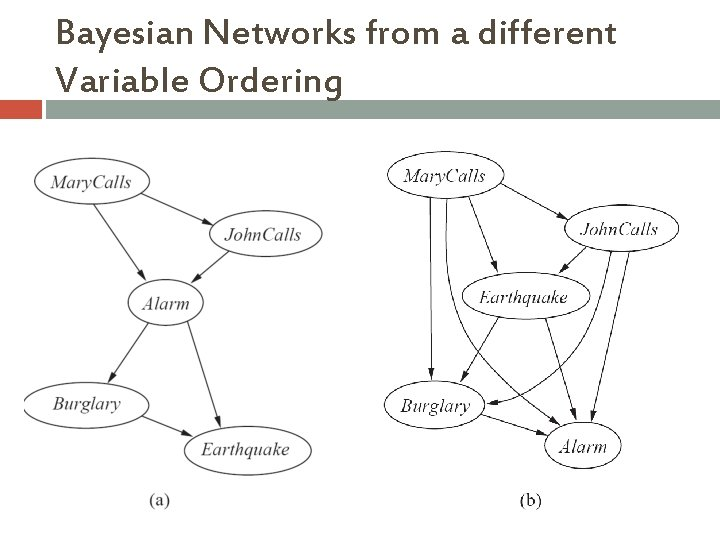Bayesian Networks from a different Variable OrderingExample for BN construction: Fire Diagnosis You want to diagnose whethere is a fire in a building You receive a noisy report about whether everyone is leaving the building If everyone is leaving, this may have been caused by a fire alarm If there is a fire alarm, it may have been caused by a fire or by tampering If there is a fire, there may be smokeExample for BN construction: Fire Diagnosis First you choose the variables. In this case, all are Boolean: Tampering is true when the alarm has been tampered with Fire is true when there is a fire Alarm is true when there is an alarm Smoke is true when there is smoke Leaving is true if there are lots of people leaving the building Report is true if the sensor reports that lots of people are leaving the building Let’s construct the Bayesian network for this � First, you choose a total ordering of the variables, let’s say: Fire; Tampering; Alarm; Smoke; Leaving; Report.Example for BN construction: Fire DiagnosisExample for BN construction: Fire DiagnosisExample for BN construction: Fire Diagnosis • Using the total ordering of variables: � Let’s say Fire; Tampering; Alarm; Smoke; Leaving; Report. • Now choose the parents for each variable by evaluating conditional independencies � � � Fire is the first variable in the ordering. It does not have parents. Tampering independent of fire (learning that one is true would not change your beliefs about the probability of the other) Alarm depends on both Fire and Tampering: it could be caused by either or both Smoke is caused by Fire, and so is independent of Tampering and Alarm given whethere is a Fire Leaving is caused by Alarm, and thus is independent of the other variables given Alarm Report is caused by Leaving, and thus is independent of the other variables given LeavingExample for BN construction: Fire Diagnosis • How many probabilities do we need to specify for this Bayesian network? • 1+1+4+2+2+2 = 12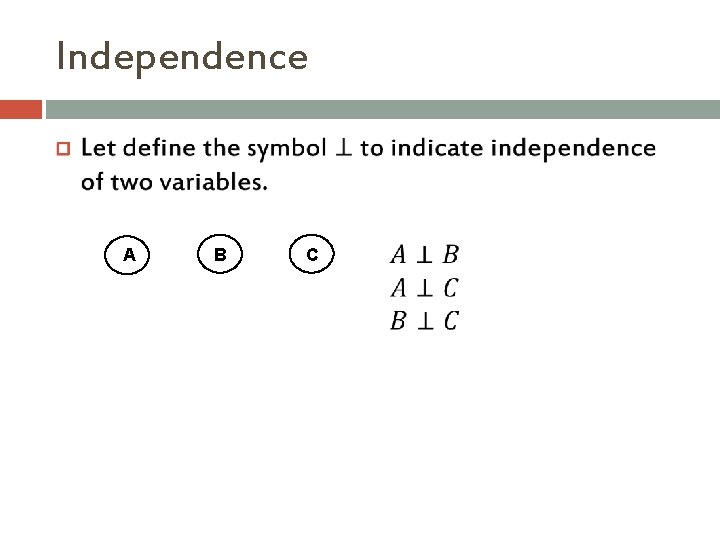Independence A B C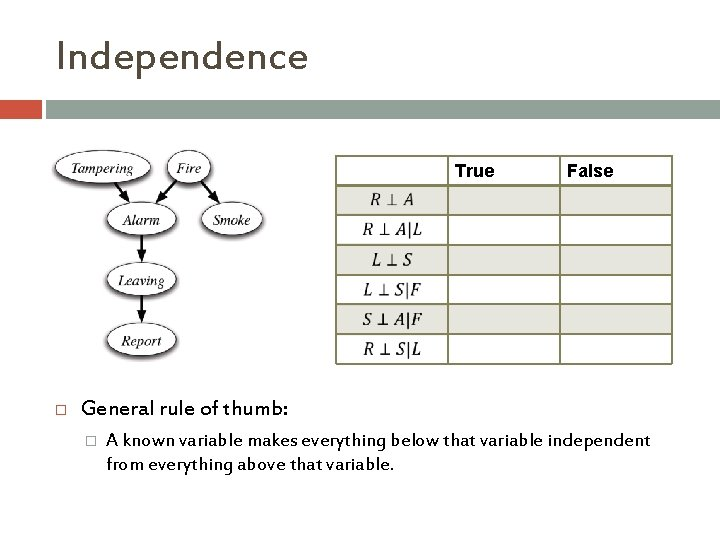Independence True False General rule of thumb: � A known variable makes everything below that variable independent from everything above that variable.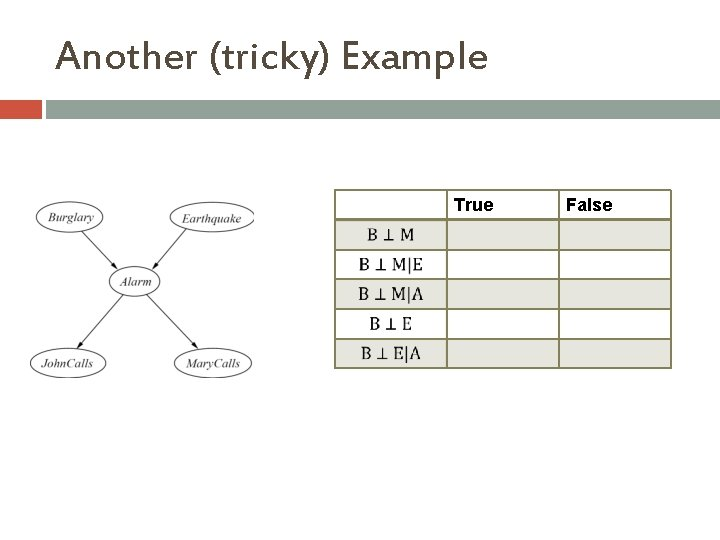Another (tricky) Example True False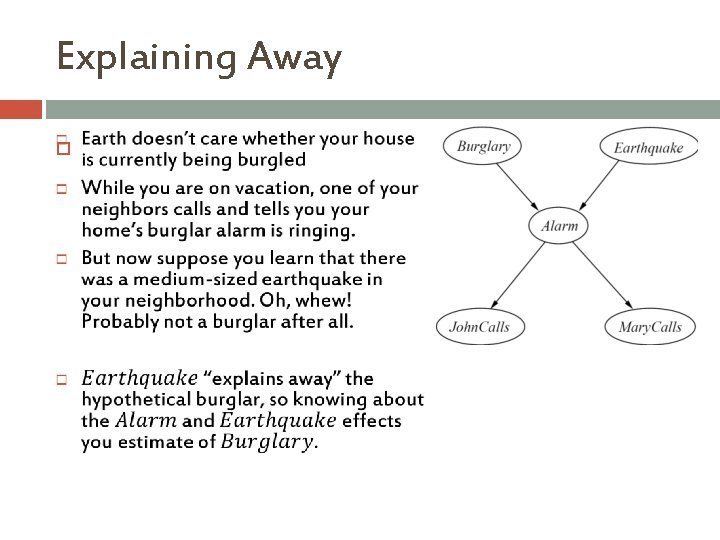Explaining AwayIndependence Is there a principled way to determine all these dependencies? � Yes! It’s called D-Separation – 3 specific rules. Some say D-separation rules are easy Our book: “rather complicated… we omit it” The truth: a mix of both… easy to state rules, can be tricky to apply. Talk to me if you want to know more.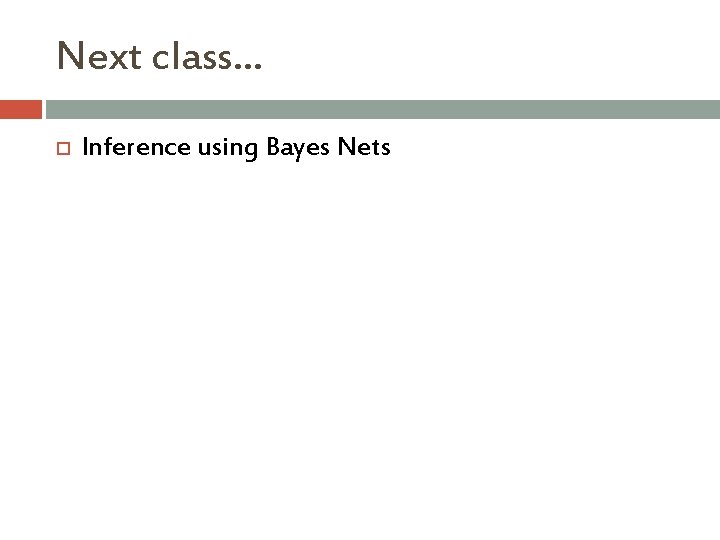Next class… Inference using Bayes Nets